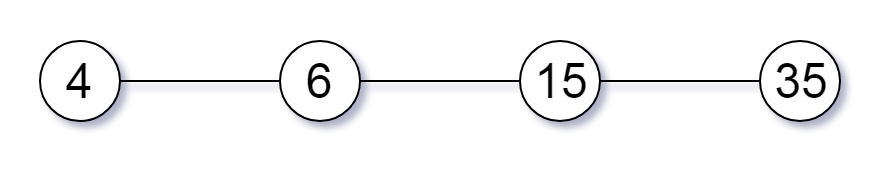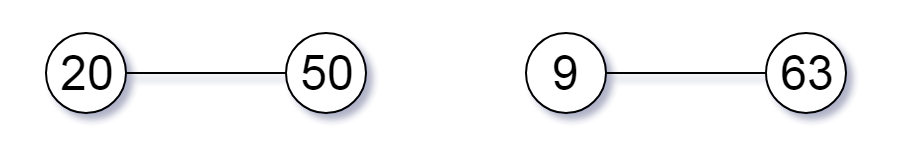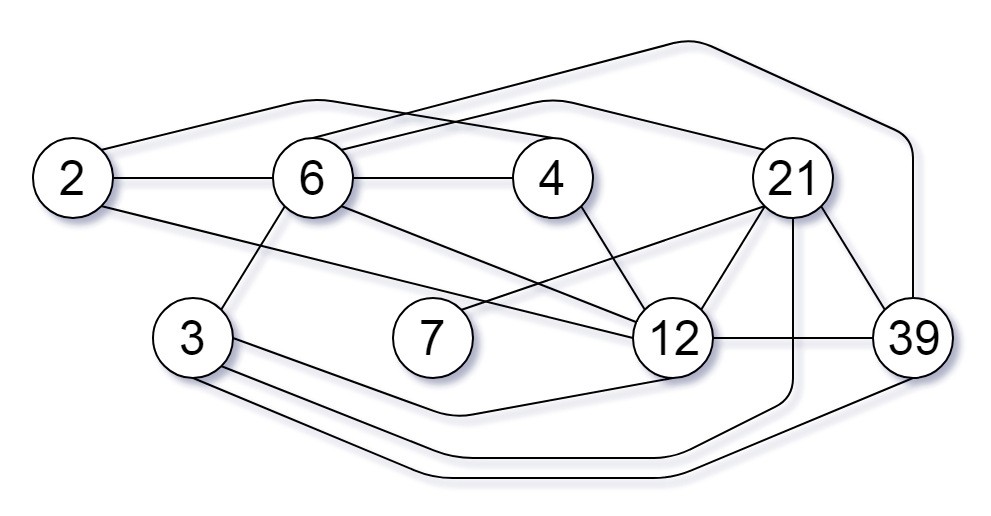## C# || How To Find The Largest Component Size By Common Factor Using C#The following is a module with functions which demonstrates how to find the largest component size by common factor using C#.

1. Largest Component Size – Problem Statement

You are given an integer array of unique positive integers nums. Consider the following graph:

• There are nums.length nodes, labeled nums to nums[nums.length – 1],
• There is an undirected edge between nums[i] and nums[j] if nums[i] and nums[j] share a common factor greater than 1.

Return the size of the largest connected component in the graph.

Example 1:``` Input: nums = [4,6,15,35] Output: 4 ```

Example 2:``` Input: nums = [20,50,9,63] Output: 2 ```

Example 3:``` Input: nums = [2,3,6,7,4,12,21,39] Output: 8 ```

2. Largest Component Size – Solution

The following is a solution which demonstrates how to find the largest component size by common factor.

The following solution uses a union find set to group connections together.

``` 2. Largest Component Size - Solution C# // ============================================================================ // Author: Kenneth Perkins // Date: Nov 28, 2021 // Taken From: http://programmingnotes.org/ // File: Solution.cs // Description: Demonstrates how to find largest common factor // ============================================================================ public class Solution { public int LargestComponentSize(int[] nums) { var set = new UnionFindSet(nums.Max() + 1); // Calculate primes set for all elements foreach (var num in nums) { for (var k = 2; k * k <= num; ++k) { if (num % k == 0) { set.Union(num, k); set.Union(num, num / k); } } } // Count the apperance of parents, return the maxium one. // All connected nodes will point to same parent var map = new Dictionary<int, int>(); var result = 1; foreach (var num in nums) { var count = set.Find(num); map[count] = (map.ContainsKey(count) ? map[count] : 0) + 1; result = Math.Max(result, map[count]); } return result; } public class UnionFindSet { private int[] parent; public UnionFindSet(int size) { parent = new int[size]; for (int i = 0; i < parent.Length; ++i) { parent[i] = i; } } public void Union(int x, int y) { parent[Find(x)] = parent[Find(y)]; } public int Find(int x) { if (parent[x] != x) { parent[x] = Find(parent[x]); } return parent[x]; } } }// http://programmingnotes.org/ 1234567891011121314151617181920212223242526272829303132333435363738394041424344454647484950515253 // ============================================================================//    Author: Kenneth Perkins//    Date:   Nov 28, 2021//    Taken From: http://programmingnotes.org///    File:  Solution.cs//    Description: Demonstrates how to find largest common factor// ============================================================================public class Solution {    public int LargestComponentSize(int[] nums) {        var set = new UnionFindSet(nums.Max() + 1);         // Calculate primes set for all elements        foreach (var num in nums) {            for (var k = 2; k * k <= num; ++k) {                if (num % k == 0) {                    set.Union(num, k);                    set.Union(num, num / k);                }            }        }         // Count the apperance of parents, return the maxium one.        // All connected nodes will point to same parent        var map = new Dictionary<int, int>();        var result = 1;        foreach (var num in nums) {            var count = set.Find(num);            map[count] = (map.ContainsKey(count) ? map[count] : 0) + 1;            result = Math.Max(result, map[count]);        }        return result;    }     public class UnionFindSet {        private int[] parent;         public UnionFindSet(int size) {            parent = new int[size];            for (int i = 0; i < parent.Length; ++i) {                parent[i] = i;            }        }        public void Union(int x, int y) {            parent[Find(x)] = parent[Find(y)];        }        public int Find(int x) {            if (parent[x] != x) {                parent[x] = Find(parent[x]);            }            return parent[x];        }    }}// http://programmingnotes.org/ ```

QUICK NOTES:
The highlighted lines are sections of interest to look out for.

The code is heavily commented, so no further insight is necessary. If you have any questions, feel free to leave a comment below.

Once compiled, you should get this as your output for the example cases:

``` 4 2 8 ```